# Calculate the Xth Weekday of Any Month in Excel

I’ve a simple formula for calculating the Xth Weekday of ANY month. It takes four inputs: Year, Month, Week, and Day. And requires a couple of lookup tables for data validation, one with special formatting.### The History

It all started when my wife mentioned there were a couple of meetings she had to schedule at work, one on the second Tuesday of every month and the other on the third Tuesday of every month. That got me to thinking about how to create a formula in Excel to calculate those dates.

After getting a partial solution, other things took precedence and it slipped from my mind until Chandoo posted a homework article on how to figure out what date Thanksgiving falls on each year, the 4th Thursday of November, in the USA.

His solution and all the variations provided in the comments (I posted one too) was focused and specific to one day (Thursday) of one week (the 4th) in one month (November). Albeit a link in that article showed another formula solution to Canada’s Thanksgiving day that takes place on the 2nd Monday of October.

This served to spur me on and finish what I started. I hope you like it.

### The Inputs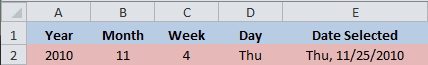Input for the Year in cell A2 is done manually. Just type in a year.

Input for Month in cell B2 is from a data validation drop-down list of values from 1-12. Merely done for convenience.

Input for Week in cell C2 is from a data validation drop-down list of values from 1-4. These are the only values that work correctly in the formula.

Input for Day in cell D2 is from a data validation drop-down list of values from 1-7 that use the “ddd” custom cell format to convert those numbers into an actual date. (You can’t actually type in a text value because what you’re seeing is a formatted date. Below I’ll show an alternate formula where you can use text values like Sun, Mon, … Sat.)

## The Formula

The formula for Date Selected in cell E2 is uses the “ddd, m/d/yyyy” custom date format (US) and the formula is:

=DATE(A2,B2,(C2*7+1)-WEEKDAY(DATE(A2,B2,8-DAY(D2))))

Instead of putting all the calculations in the Day argument of the DATE function, you can also write the formula as DATE – WEEKDAY:

=DATE(A2,B2,(C2*7+1))-WEEKDAY(DATE(A2,B2,8-DAY(D2)))

### The Explanation – Why This Works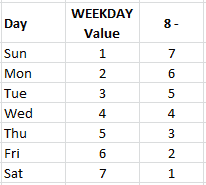This formula has two parts, subtracting the WEEKDAY from the DATE. They both hinge on the fact that WEEKDAY function values are 1-7 for days Sun-Sat, and that an inverse relationship exists for the first week of the month, as shown by the chart. (Eight minus the WEEKDAY value)

Column three is the Day value, or 3rd argument, of the DATE function inside the WEEKDAY function. This relationship works for every day of the week. For a Tuesday, we have WEEKDAY(DATE(Year, Month, 5)). For a Thursday we have WEEKDAY(DATE(Year, Month, 3)).

The DATE Function relies on the Week number 1-4 to generate a date that is one day past that particular week. C2 * 7 +1, is just giving values 8, 15, 22, or 29.

So for a Thursday Thanksgiving in the USA you have

DATE(Year, 11, 29 – WEEKDAY(DATE(Year, 11, 3)))

and for a Tuesday Thanksgiving in Canada you have

DATE(Year, 10, 15 – WEEKDAY(DATE(Year, 10, 6)))

It helps to visualize this formula in the Evaluate Formula dialog box and step through the formula iteration.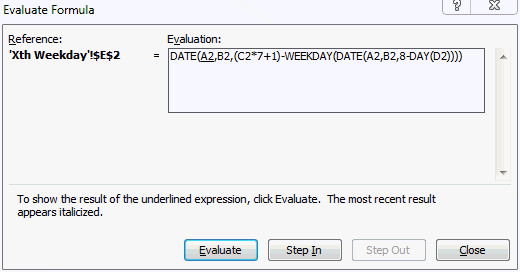### The Data Validation

One critical aspect of this formula is to get a numerical date value from cell D2 for the DAY function. I use values 1-7 and convert them to Dates by custom formatting the cells with the “ddd” format.Of course this resulted in my date range being 1 Jan, 1900 to 7 Jan, 1900 and is okay for the Windows Date System.

Note: Excel for Mac users check to make sure you’re not using the 1904 Date System, in Excel>Preferences>Calculation look under the Workbook options to see if Use the 1904 date system is unchecked.

If you want to make this work with either date system (Windows or Mac) then choose a month where Sunday is the first day, like 1 Aug, 2010. You’ll need seven dates ending with 7 Aug, 2010.

## Alternate Formula Without Custom Formatting

You can, of course, skip the custom formatting for the Day in cell D2 by using straight text values in the data validation list.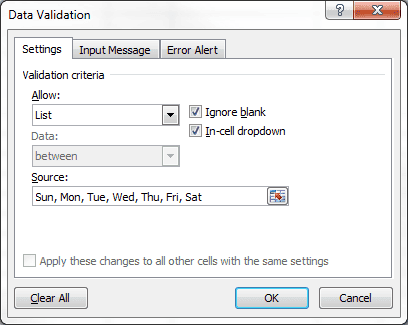This requires a different formula and below I’m utilizing the VLOOKUP function.

=DATE(A2,B2,(C2*7+1)-WEEKDAY(DATE(A2,B2,VLOOKUP(D2,{“Sun”,7;”Mon”,6;”Tue”,5;”Wed”,4;”Thu”,3;”Fri”,2;”Sat”,1},2,FALSE))))

The VLOOKUP reads cell D2 then finds the corresponding value in the constant array and returns a number.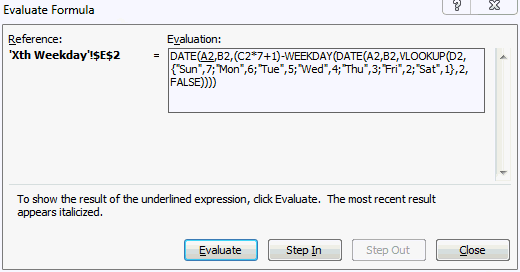To shorten this up considerably, create a Named Constant, like MyWeekday, to replace the array.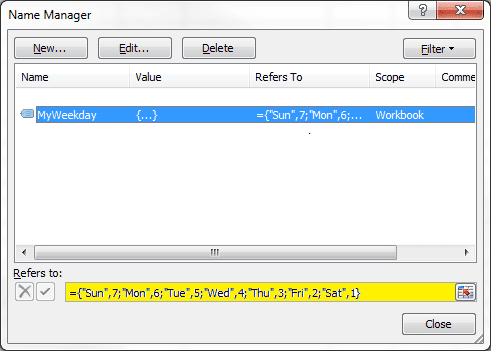The formula can then be reduced to:

=DATE(A2,B2,(C2*7+1)-WEEKDAY(DATE(A2,B2,VLOOKUP(D2,MyWeekday,2,FALSE))))

I’m sure there are other variations. Do you have a better solution?

XLSX File Format: Excel 2007, 2010, 2011

XLS File Format: Excel 2003, 2008

Here’s another file with the alternate formula using the VLOOKUP with constant array.

XLSX File Format: Excel 2007, 2010, 2011

XLS File Format: Excel 2003, 2008## 5 thoughts on “Calculate the Xth Weekday of Any Month in Excel”

1. Pingback: Tweets that mention Calculate the Xth Weekday of Any Month in Excel | Excel Semi-Pro -- Topsy.com

2.Andrew Burns

I was looking to use your formula here to build a data array of economic data. Some economic data, such as employment statistics, are released on the first Friday of a month. My question is, say I had a vector in excel (single column) of julian dates (formatted into any date format I like), how can I alter your formula such that it gives me a value from 1 to 5? In other words, today, 01/16/13, would be a “3”, for the 3rd Wednesday of January 2013, etc., etc.

Thanks!

1.Gregory

What you are looking for is the week number of the month and the weekday. My formula won’t give you that and neither will a variation of that formula.

I’m not sure how you would do this exactly. I tried to use the WEEKNUM formula to get the week of the year, but this is a cumulative number that ends in December at week 52 or 53, so you don’t get 1 to 5 for each month. I tried subtracting the week number from the previous month’s end date, but that doesn’t work in January and there are weeks that spilt the end of month so you have to specify the day of the week as well.

Perhaps I’m taking the wrong approach, but the short of it is I haven’t figure out this problem yet. Sorry.

1.Andrew Burns

I’m thinking we have to someone divide the date by 7 then round it using INT to get the week that date of the month is in? Then also using the first Monday of every month would always be either the first or second week of a month? Some smart combination of these things …

3.Scott

Thankyou so much, This has been such a help for me. I have learned so much too